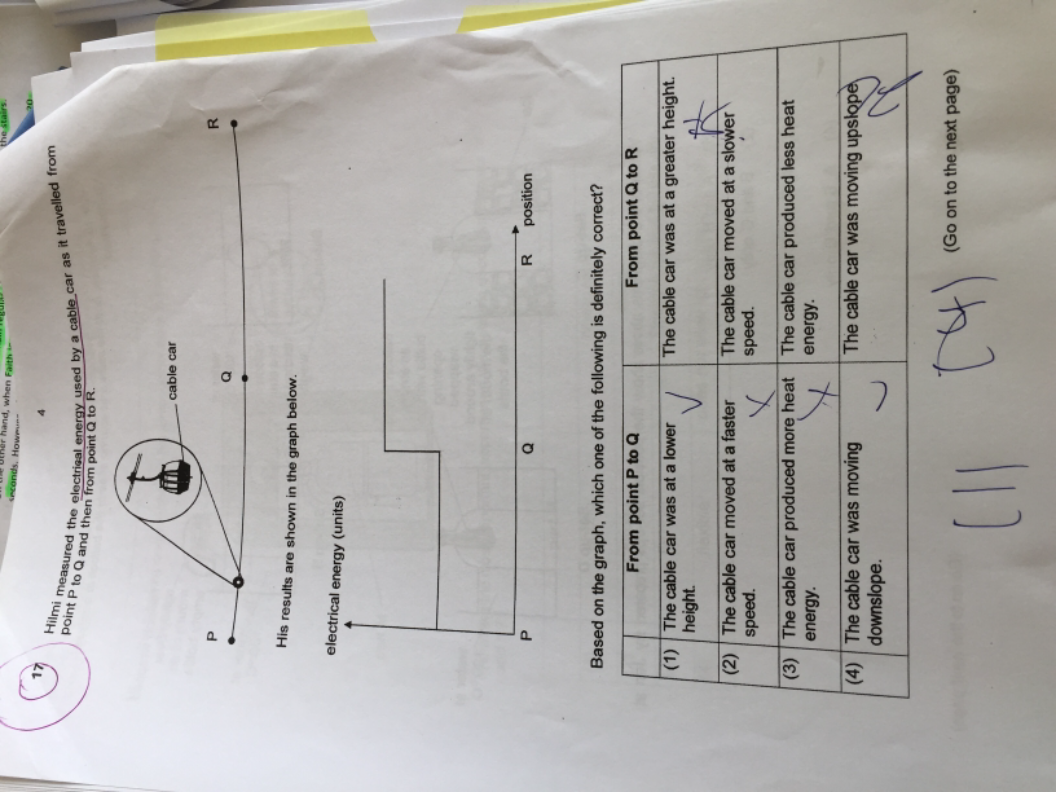# QuestionHi can help me with this question? Answer sheet is 4 but I thought the answer should be 1. Thks

1 Answer

# Answer

I think the answer (4) is correct as the amount of energy is determined by the amount of work done.

From point P to Q

• If the cable car is moving downslope, it needs less energy => the amount of electrical energy needed is lower

From point Q to R

• More electrical energy is needed if the cable car is moving upslope.
• Even if it is at a greater height, it is simply “higher” but could still be traveling horizontally. Therefore, the amount of energy being used to move it would be the same as if the cable car is travelling horizontally at any height.
• However, what you are probably thinking of is that it has greater gravitational potential energy (since it is at a greater height). But it doesnt mean that the cable car needs more energy to continue moving.
1 Reply 0 Likes ✔Accepted Answer

Thks!

0 Replies 0 Likes